Question

Suppose that a 9-year bond with a face value of 1000 dollars pays semiannual coupons at a rate of 5.2 percent per half year. The issuer of the bond has the option to redeem it at the time of the 16th coupon for 2100 dollars, or at maturity for 2000 dollars. Find the price that will guarantee an investor a yield rate of at least 12.3 percent convertible semiannually, regardless of when the bond is redeemed.

Price when redeemed at 16th coupon:

Nper = 16
FV = 2100
PMT = 1000 * 5.2% = 52
Rate = 12.3%/2 = 6.15%

Bond price can be calculated by using the following excel formula:
=PV(rate,nper,pmt,fv)
=PV(6.15%,16,-52,-2100)
= \$1,328.30

Price when redeemed at 16th coupon = \$1,328.30.

Price when redeemed at maturity:

FV = 2000
Nper = 9 * 2 = 18
PMT = 52
Rate = 6.15%

Bond price can be calculated by using the following excel formula:
=PV(rate,nper,pmt,fv)
=PV(6.15%,18,-52,-2000)
= \$1,239.83

Price when redeemed at maturity = \$1,239.83

#### Earn Coins

Coins can be redeemed for fabulous gifts.

Similar Homework Help Questions
• ### (1 point) Suppose that a 9-year bond with a face value of 1000 dollars pays semiannual...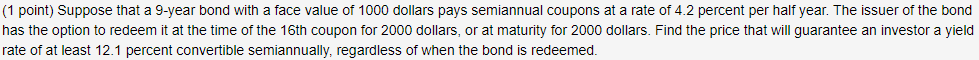(1 point) Suppose that a 9-year bond with a face value of 1000 dollars pays semiannual coupons at a rate of 4.2 percent per half year. The issuer of the bond has the option to redeem it at the time of the 16th coupon for 2000 dollars, or at maturity for 2000 dollars. Find the price that will guarantee an investor a yield rate of at least 12.1 percent convertible semiannually, regardless of when the bond is redeemed.

• ### (1 point) A 9-year bond with a face value of 1000 dollars is redeemable at par,...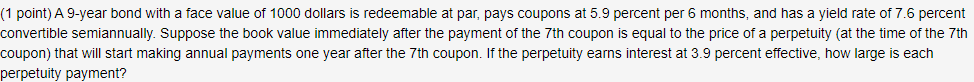(1 point) A 9-year bond with a face value of 1000 dollars is redeemable at par, pays coupons at 5.9 percent per 6 months, and has a yield rate of 7.6 percent convertible semiannually. Suppose the book value immediately after the payment of the 7th coupon is equal to the price of a perpetuity (at the time of the 7th coupon) that will start making annual payments one year after the 7th coupon. If the perpetuity earns interest at 3.9...

• ### A coupon bond with a par value of \$1,000 and a 10-year maturity pays semiannual coupons...

A coupon bond with a par value of \$1,000 and a 10-year maturity pays semiannual coupons of \$21. (a) Suppose the yield for this bond is 4% per year compounded semiannually. What is the price of the bond? (b) Is the bond selling above or below par value? Why?

• ### 5. A 30-year 1000 par value bond with coupons at 9% payable semiannually and a redemption...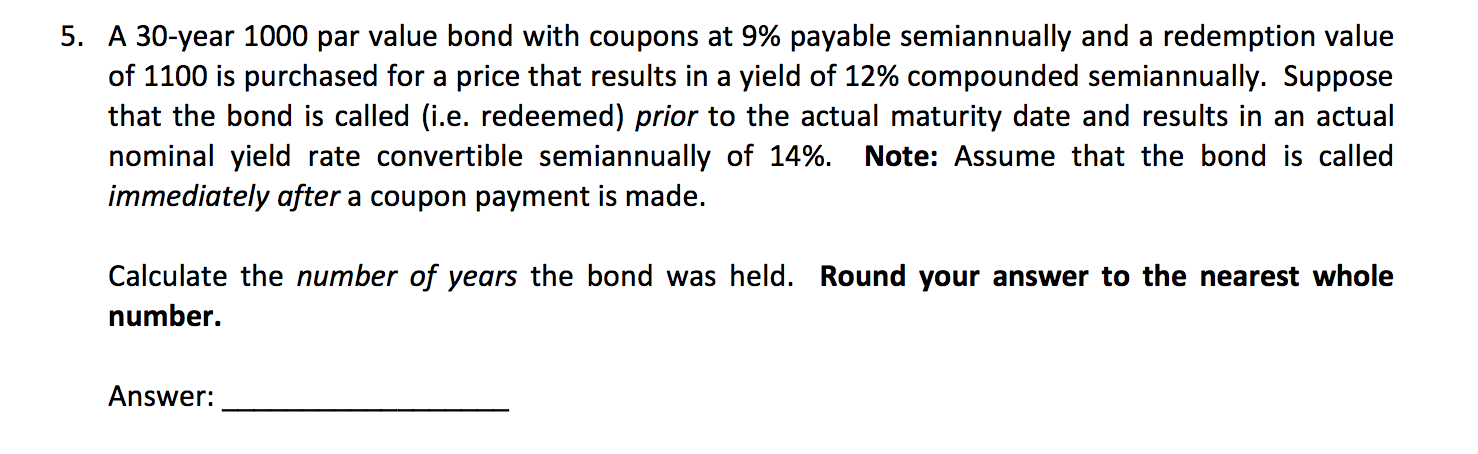5. A 30-year 1000 par value bond with coupons at 9% payable semiannually and a redemption value of 1100 is purchased for a price that results in a yield of 12% compounded semiannually. Suppose that the bond is called (i.e. redeemed) prior to the actual maturity date and results in an actual nominal yield rate convertible semiannually of 14%. Note: Assume that the bond is called immediately after a coupon payment is made. Calculate the number of years the bond...

• ### (1 point) A 13-year bond with a face value of 2000 dollars earns interest at 9.2...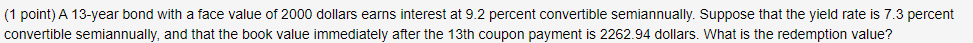(1 point) A 13-year bond with a face value of 2000 dollars earns interest at 9.2 percent convertible semiannually. Suppose that the yield rate is 7.3 percent convertible semiannually, and that the book value immediately after the 13th coupon payment is 2262.94 dollars. What is the redemption value?

• ### 4.1.5 Don purchases a 1000 par value 10-year bond with 8% semiannual coupons for 900. He...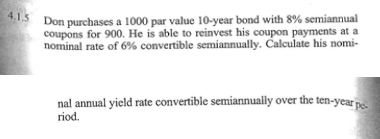4.1.5 Don purchases a 1000 par value 10-year bond with 8% semiannual coupons for 900. He is able to reinvest his coupon payments at a nominal rate of 6% convertible semiannually. Calculate his nomi- nal annual yield rate convertible semiannually over the ten-year riod.

• ### (1 point) Two 1000 dollar face value bonds are both redeemable at par, with the first...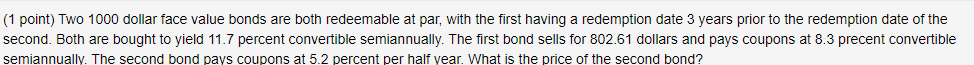(1 point) Two 1000 dollar face value bonds are both redeemable at par, with the first having a redemption date 3 years prior to the redemption date of the second. Both are bought to yield 11.7 percent convertible semiannually. The first bond sells for 802.61 dollars and pays coupons at 8.3 precent convertible semiannually. The second bond pays coupons at 5.2 percent per half year. What is the price of the second bond?

• ### 15. A 10-year 1000 par value bond was purchased to yield 6% convertible semiannually. The bond...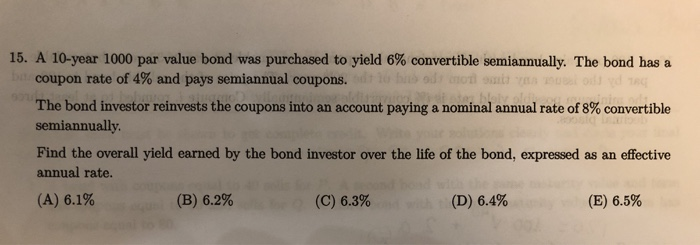15. A 10-year 1000 par value bond was purchased to yield 6% convertible semiannually. The bond has a bucoupon rate of 4% and pays semiannual coupons. The bond investor reinvests the coupons into an account paying a nominal annual rate of 8% convertible semiannually. Find the overall yield earned by the bond investor over the life of the bond, expressed as an effective annual rate. (A) 6.1% (B) 6.2% (C) 6.3% (D) 6.4% (E) 6.5%

• ### (1 point) A 7-year bond with a face value of 5000 dollars is redeemable at par...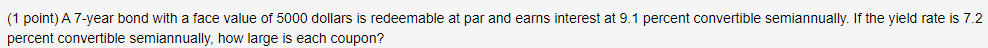(1 point) A 7-year bond with a face value of 5000 dollars is redeemable at par and earns interest at 9.1 percent convertible semiannually. If the yield rate is 7.2 percent convertible semiannually, how large is each coupon?

• ### A 1000 par-value 15-year bond has semiannual coupons of 60 each. This bond is callable at any of the last 10 coupon dat...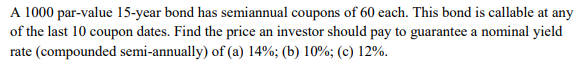A 1000 par-value 15-year bond has semiannual coupons of 60 each. This bond is callable at any of the last 10 coupon dates. Find the price an investor should pay to guarantee a nominal yield rate (compounded semi-annually) of (a) 14%; (b) 10%; (c) 12%.